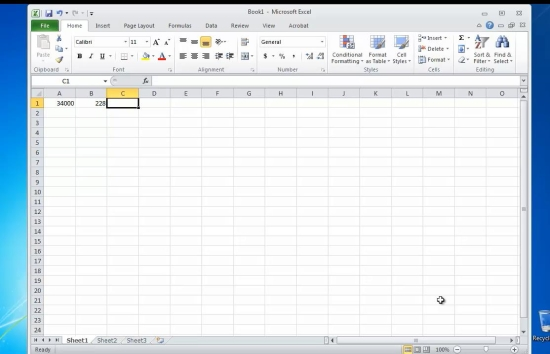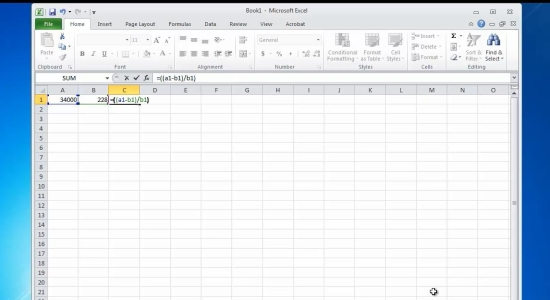## How to Calculate Percent Change in Excel

In this tutorial you will learn how to calculate percent change in Excel

Percent change, or percentile change, is a statistic indicator that shows the growth or decline between two values. It is also known as the rate of change or percent of growth rate.

The formula for percent change is:

Pc = {(A-B)/B} x 100, where A is the first value, and B is the second value. Here is how you calculate it in Excel.

Prerequisites: Microsoft Excel.

Step 1. Launch Excel and open the document that needs to calculate percentile change.

Step 2. Enter the two values in two separate cells.Step 3. Position the cursor to the cell that you want to hold the result.

Type the following:

=((AAxx – BBxx)/BBxx)*100, where

AAxx and BBxx are the two values that you want to calculate the percentage change to.

Hit Enter. Excel will do the calculation and will display the result.Result: Congratulations! You have learnt how to calculate percent change in Excel.